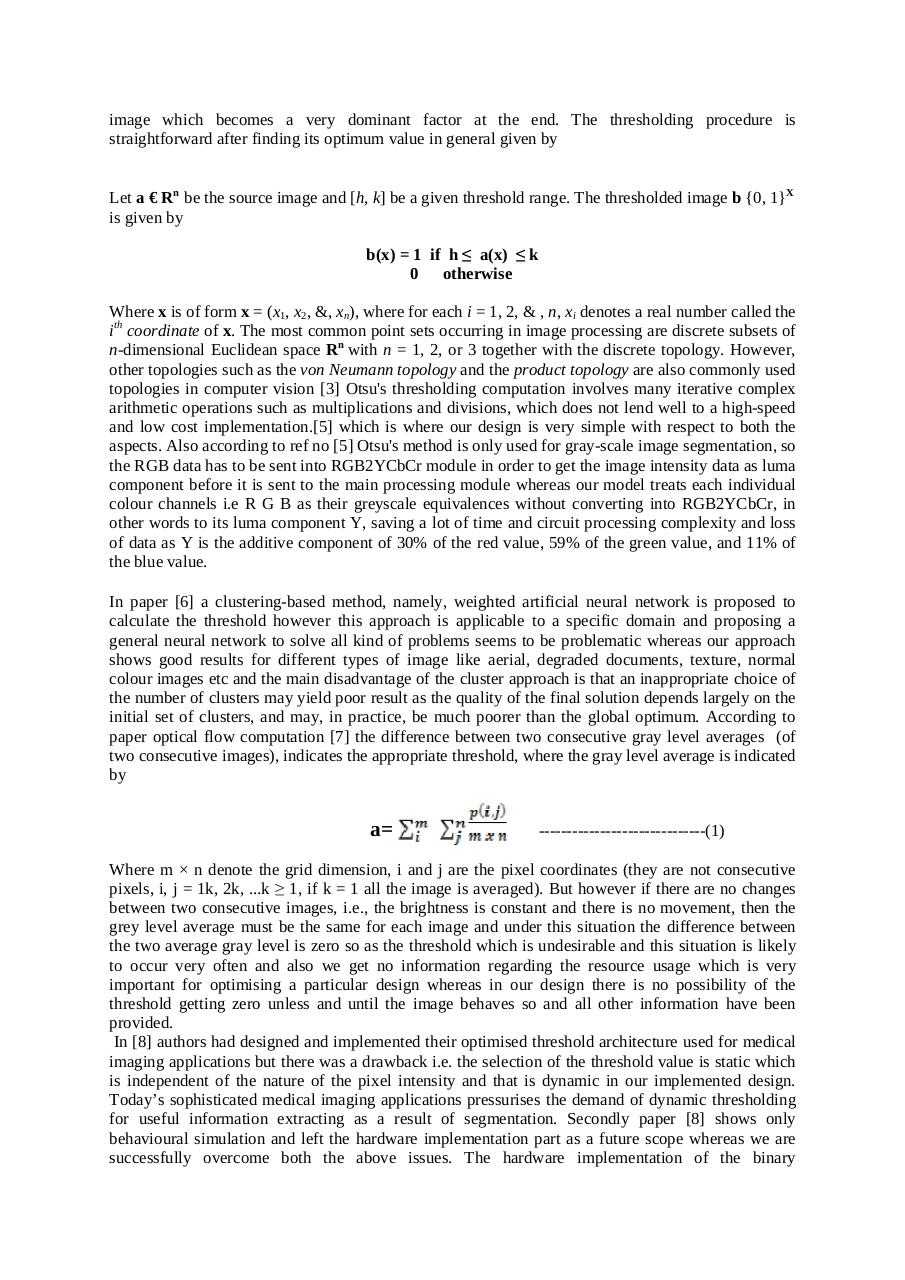# image processing and applications on Cryptography.pdfPage 1 2 3 4 5 6 7 8 9 10

#### Text preview

image which becomes a very dominant factor at the end. The thresholding procedure is
straightforward after finding its optimum value in general given by
Let a € Rn be the source image and [h, k] be a given threshold range. The thresholded image b {0, 1}X
is given by
b(x) = 1 if h ≤ a(x) ≤ k
0
otherwise
Where x is of form x = (x1, x2, &amp;, xn), where for each i = 1, 2, &amp; , n, xi denotes a real number called the
ith coordinate of x. The most common point sets occurring in image processing are discrete subsets of
n-dimensional Euclidean space Rn with n = 1, 2, or 3 together with the discrete topology. However,
other topologies such as the von Neumann topology and the product topology are also commonly used
topologies in computer vision  Otsu's thresholding computation involves many iterative complex
arithmetic operations such as multiplications and divisions, which does not lend well to a high-speed
and low cost implementation. which is where our design is very simple with respect to both the
aspects. Also according to ref no  Otsu's method is only used for gray-scale image segmentation, so
the RGB data has to be sent into RGB2YCbCr module in order to get the image intensity data as luma
component before it is sent to the main processing module whereas our model treats each individual
colour channels i.e R G B as their greyscale equivalences without converting into RGB2YCbCr, in
other words to its luma component Y, saving a lot of time and circuit processing complexity and loss
of data as Y is the additive component of 30% of the red value, 59% of the green value, and 11% of
the blue value.
In paper  a clustering-based method, namely, weighted artificial neural network is proposed to
calculate the threshold however this approach is applicable to a specific domain and proposing a
general neural network to solve all kind of problems seems to be problematic whereas our approach
shows good results for different types of image like aerial, degraded documents, texture, normal
colour images etc and the main disadvantage of the cluster approach is that an inappropriate choice of
the number of clusters may yield poor result as the quality of the final solution depends largely on the
initial set of clusters, and may, in practice, be much poorer than the global optimum. According to
paper optical flow computation  the difference between two consecutive gray level averages (of
two consecutive images), indicates the appropriate threshold, where the gray level average is indicated
by

a=

------------------------------(1)

Where m × n denote the grid dimension, i and j are the pixel coordinates (they are not consecutive
pixels, i, j = 1k, 2k, ...k ≥ 1, if k = 1 all the image is averaged). But however if there are no changes
between two consecutive images, i.e., the brightness is constant and there is no movement, then the
grey level average must be the same for each image and under this situation the difference between
the two average gray level is zero so as the threshold which is undesirable and this situation is likely
to occur very often and also we get no information regarding the resource usage which is very
important for optimising a particular design whereas in our design there is no possibility of the
threshold getting zero unless and until the image behaves so and all other information have been
provided.
In  authors had designed and implemented their optimised threshold architecture used for medical
imaging applications but there was a drawback i.e. the selection of the threshold value is static which
is independent of the nature of the pixel intensity and that is dynamic in our implemented design.
Today’s sophisticated medical imaging applications pressurises the demand of dynamic thresholding
for useful information extracting as a result of segmentation. Secondly paper  shows only
behavioural simulation and left the hardware implementation part as a future scope whereas we are
successfully overcome both the above issues. The hardware implementation of the binary